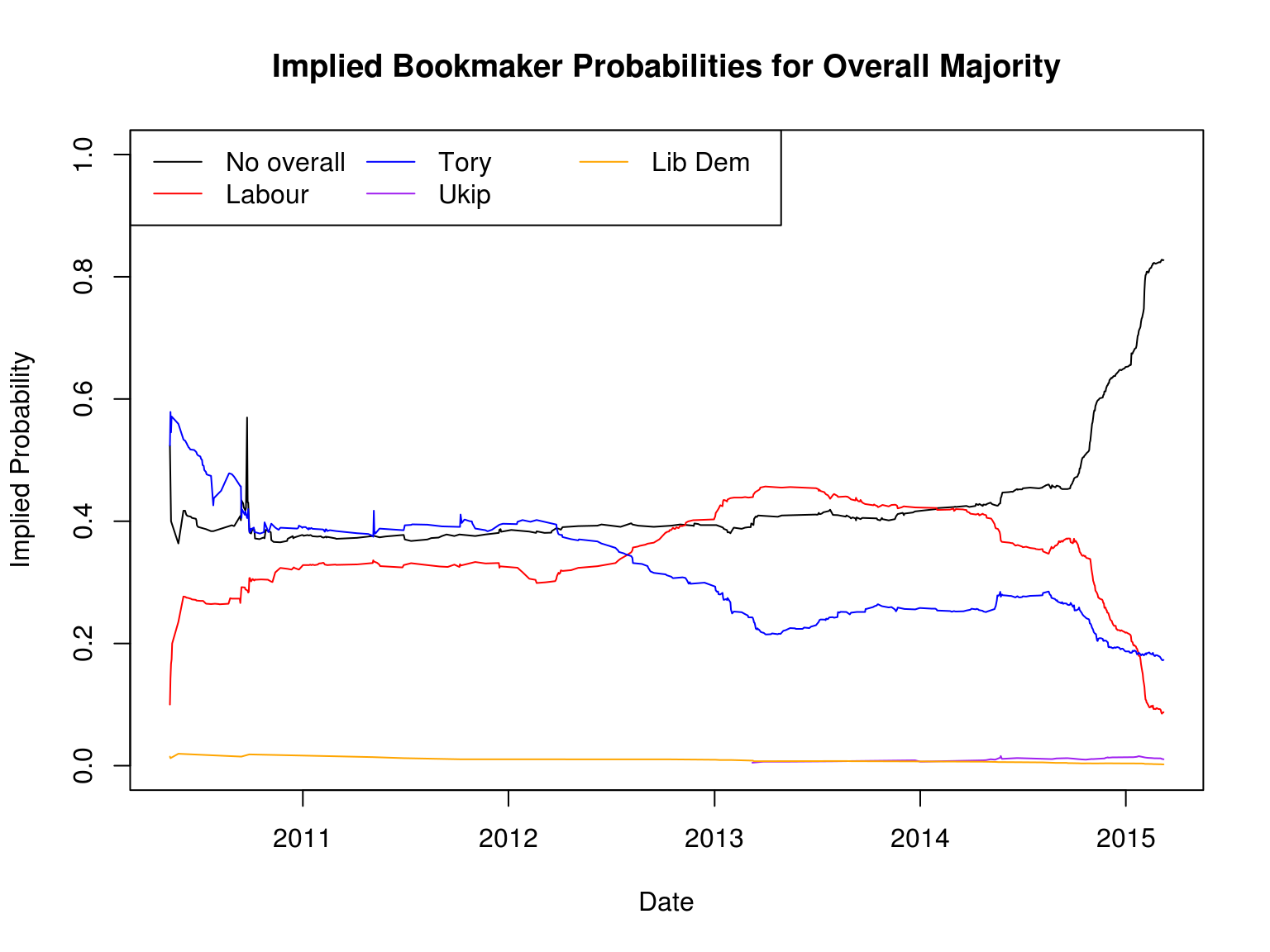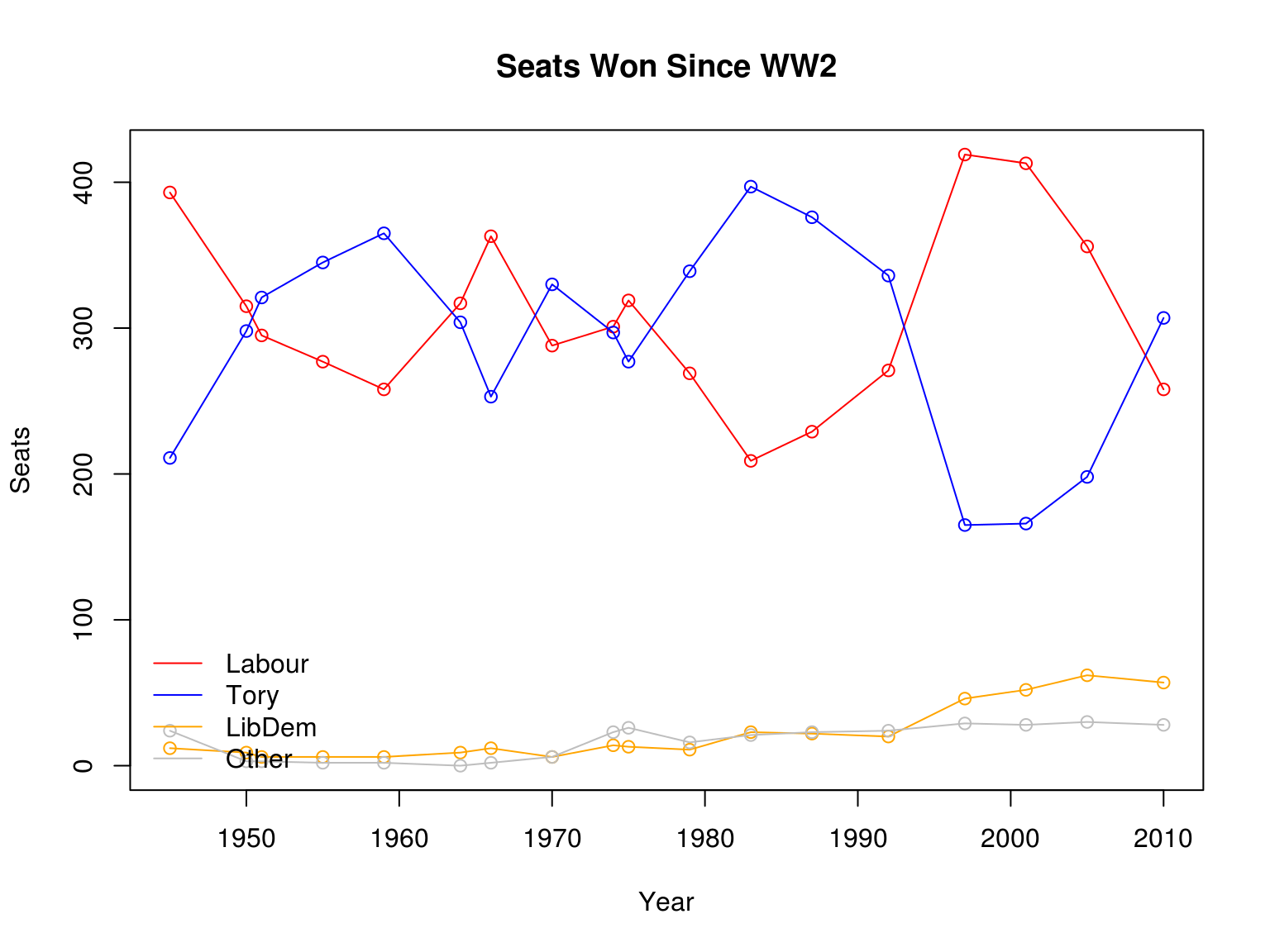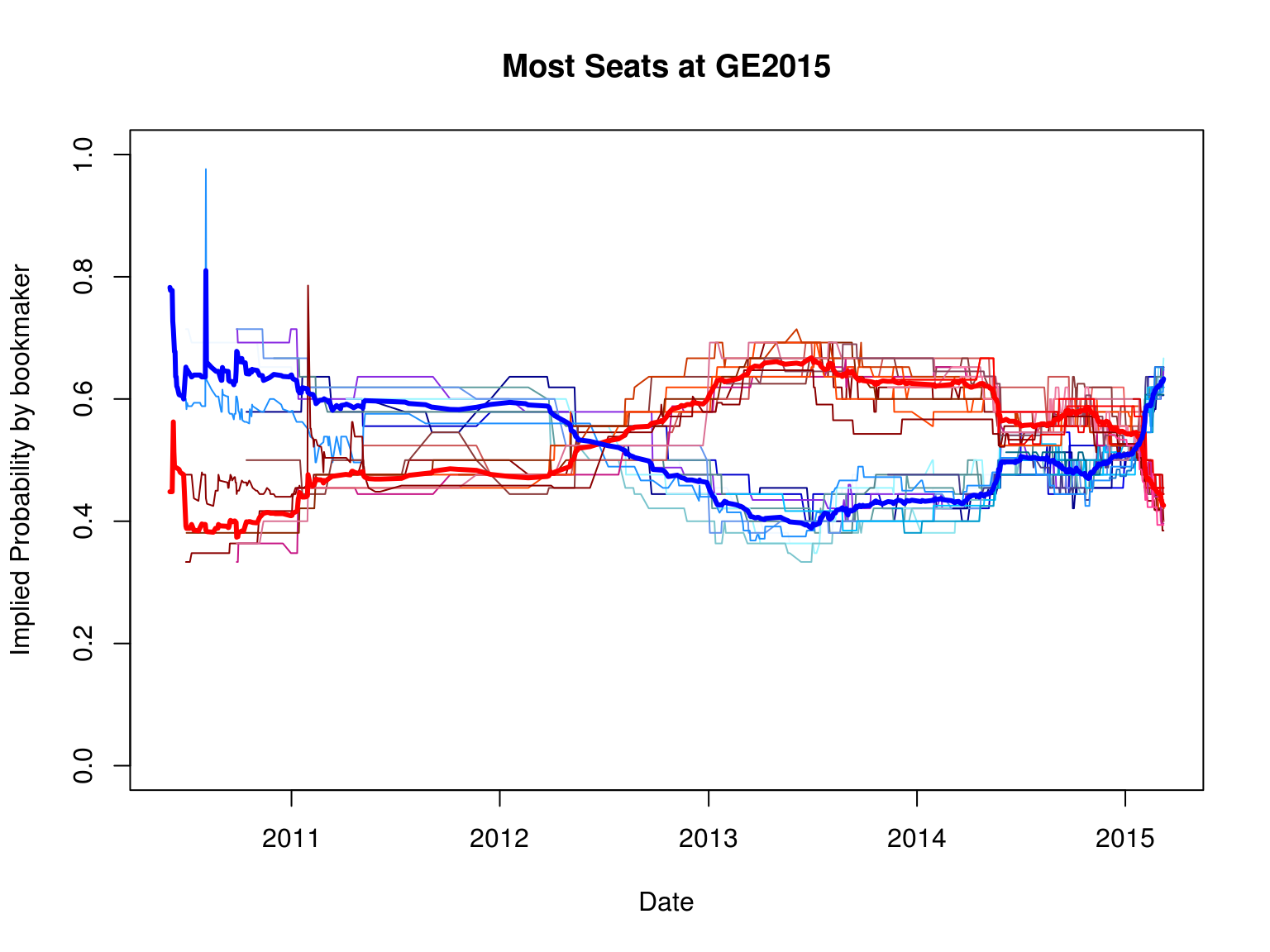10/03/2015

# Introduction

• Midterm II: Review.
• Projects:
• Presentations:

# Midterm II Review

• Marks: Emails will contain provisional mark.
• Time pressure deliberate: Vital skill for real world.
• Will it harm your degree classification? No.
• Worth 3% of it.
• Exam is moderated.
• My aim is to give you mark you deserve, no better or worse.
• Did I provide you with enough material for exam?
• Yes — all lecture slides bar first two lectures full of code.
• That I didn’t have time to cover everything does not mean you could not look over it in your own time.
• Even had I managed to go through every line in every slide, you should still be doing that anyway.
• Some minor tips:
• Use \$ to plot variables rather than entire datasets.
• Breakdown code into all its parts, work out what each does.
• Strategy for learning coding:
• Copy and paste code from textbook and my slides.
• Check it works.
• Amend it for your purposes.

# Project: Details

• Develop a model to forecast a variable of your choice.
• At least one event must happen after deadline.
• Details:
• Weight: 40% of final mark.
• Word count: 5000 words.
• Presentation:
• Date: 25 March.
• Weight: 10% of final mark.
• Length: 5 minutes absolute maximum.
• Recorded for moderation purposes.
• Structure:
• Introduction: What I am going to do.
• Why should a reader be interested?
• What is the important/relevant context?
• Main Part: Do it.
• Literature review: Who else has done anything like this?
• Methodology: How I’ll do it.
• Data: What data I’m using (collection, properties, characteristics).
• Results: What I’ve done (tables, plots, all models, compare and contrast)
• Conclusions: Here’s what I did.
• Review what you did in less than a paragraph.
• Do not introduce anything new.
• Recommendation:
• Use RStudio for slides for talk and overall project.

# Project: Steps

• Make it something you’re really interested in.
• Talk to me about it, particularly if speculative.
• Can you collect data?
• Does your variable satisfy properties set out in lecture 1?
3. Start constructing forecast models.
• What are the properties of your data?
• What particular features of your data do you need to account for? How will you do it?
• What forecast models are most appropriate/inappropriate?

# What about Lectures and Classes?

• Lectures:
• I’ll develop an ambitious project idea:
• Forecasting the General Election.
• Class tomorrow:
• Covering whatever we didn’t manage to cover today.
• Class in week 10:
• Your call: Come along if you want.
• Email in advance with topics you’d like covered.
• If no topics received, same format as lecture.
• Class slot in week 11:
• Presentations.

# Forecasting the General Election

• Ambitious project idea (does not mean you cannot also do election: talk to me).
• General Election occurs on May 7:
• Arguably most unpredictable election ever!
• R code notes:
• `clean.data` is a `function`, as in it’s a collection of commands.
• Functions useful to avoid needing to copy and paste endlessly the same commands.
• An example of a function could be as simple as `load.my.data` which takes as an argument where your data is, and adds the command `stringsAsFactors=F` line for you each time. This would look like:
``````load.my.data <- function(loc) {
return(data)
}``````
• We’d then use it like the following:
``````loc0 <- "/home/readejj/Dropbox/Research/Misc/LVW Projects/UK Elections/data/"
loc <- paste(loc0,"seats_1945_2010.csv",sep="")
print(loc)``````
``##  "/home/readejj/Dropbox/Research/Misc/LVW Projects/UK Elections/data/seats_1945_2010.csv"``
``all.outcomes <- load.my.data(loc)``
• Hence first part of following code sets out what this function is:
• It cleans up a dataset which, when opened, looks quite messy.
• Opened file is in html, which is highly regular hence can be cleaned up by set of commands.
``````clean.data <- function(data) {
require(zoo)
colnames(data) <- "Date.Time"
colnames(data)[-1] <- gsub("^.*?\\d+_(\\w+)[.]href.*?\$","\\1",colnames(data)[-1])
data <- data[,-NCOL(data)]
data\$Date.Time <- as.Date(substring(data\$Date.Time,2),"%Y-%m-%d")
data <- data[is.na(data\$Date.Time)==F,]
data <- data[order(data\$Date.Time),]
for(i in colnames(data)[nchar(colnames(data))==2]) {
data[,i] <- gsub("<div class=.*?>(.*?)</div>","\\1",data[,i])
data[,i] <- gsub("<div class=.*?>(.*?)\\s+\$","\\1",data[,i])
data[,i] <- gsub(">","",data[,i])
data[,i] <- gsub("^\\s+|\\s+\$","",data[,i])
#need to turn fractional odds into decimal odds
numerator <- gsub("(\\d+)/(\\d+)\$","\\1",data[,i])
denominator <- gsub("(\\d+)/(\\d+)\$","\\2",data[,i])
data[,i] <- as.numeric(numerator)+1
data[numerator!=denominator,i] <- as.numeric(numerator[numerator!=denominator])/as.numeric(denominator[numerator!=denominator])+1
data[,i] <- na.locf(data[,i],na.rm=F)
}
return(data)
}``````
• Having set out the function, next we open up data on beting prices for the general election:
• The messy files mentioned above.
``````loc <- "/home/readejj/Dropbox/Research/Misc/LVW Projects/UK Elections/data/betting/2015-03-09/"
markets <- dir(loc) #this command lists all files/folders in a location

loc3 <- paste(loc,markets,sep="") #here I'm taking just the third market, overall-majority
maj.types <- dir(loc3,pattern="*.csv") #getting all files that end in .csv
maj.type <- data.frame() #setting up empty data frame to fill up with the contents of the files we're
#going to open; idea is we want all data on different party majorities in same file
for(i in maj.types) { #look on the different files
temp <- clean.data(temp) #clean up the data using the function above
temp\$maj.typ <- gsub("-majority[.]csv","",i) #create a variable that says what kind of majority this file is for
maj.type <- rbind(maj.type,temp) #append data on the bottom of the existing dataset
}``````
``````## Loading required package: zoo
##
## Attaching package: 'zoo'
##
## The following objects are masked from 'package:base':
##
##     as.Date, as.Date.numeric``````
``## Warning: NAs introduced by coercion``
``## Warning: NAs introduced by coercion``
``## Warning: NAs introduced by coercion``
``## Warning: NAs introduced by coercion``
``## Warning: NAs introduced by coercion``
``## Warning: NAs introduced by coercion``
``## Warning: NAs introduced by coercion``
``## Warning: NAs introduced by coercion``
``## Warning: NAs introduced by coercion``
``## Warning: NAs introduced by coercion``
``## Warning: NAs introduced by coercion``
``## Warning: NAs introduced by coercion``
``## Warning: NAs introduced by coercion``
``## Warning: NAs introduced by coercion``
``## Warning: NAs introduced by coercion``
``## Warning: NAs introduced by coercion``
``## Warning: NAs introduced by coercion``
``## Warning: NAs introduced by coercion``
``## Warning: NAs introduced by coercion``
``## Warning: NAs introduced by coercion``
``## Warning: NAs introduced by coercion``
``## Warning: NAs introduced by coercion``
``## Warning: NAs introduced by coercion``
``## Warning: NAs introduced by coercion``
``## Warning: NAs introduced by coercion``
``## Warning: NAs introduced by coercion``
``## Warning: NAs introduced by coercion``
``## Warning: NAs introduced by coercion``
``## Warning: NAs introduced by coercion``
``## Warning: NAs introduced by coercion``
``## Warning: NAs introduced by coercion``
``## Warning: NAs introduced by coercion``
``## Warning: NAs introduced by coercion``
``## Warning: NAs introduced by coercion``
``## Warning: NAs introduced by coercion``
• Our data is now loaded up; running `summary(maj.type)` will give information, or perhaps better `str(maj.type)`.
• We can do all sorts with this dataset; for now, let’s look at the implied probabilities.
• With traditional odds (3-to-1 etc), we can turn them into implied probabilities.
• Treat traditional odds as fraction, so 3-to-1 is 3, but 3-to-1 on is 1/3.
• Add 1 to the traditional odds fractionised, this gives decimal odds.
• Take reciprocal of decimal odds to get implied probability.
``````maj.type\$mean <- rowMeans(maj.type[,nchar(colnames(maj.type))==2],na.rm=T)
plot(maj.type\$Date.Time[maj.type\$maj.typ=="no-overall"],1/maj.type\$mean[maj.type\$maj.typ=="no-overall"],
ylim=range(0,1),xlim=range(maj.type\$Date.Time),type="l",ylab="Implied Probability",xlab="Date",
main="Implied Bookmaker Probabilities for Overall Majority")
lines(maj.type\$Date.Time[maj.type\$maj.typ=="labour"],1/maj.type\$mean[maj.type\$maj.typ=="labour"],col="red")
lines(maj.type\$Date.Time[maj.type\$maj.typ=="conservative"],1/maj.type\$mean[maj.type\$maj.typ=="conservative"],
col="blue")
lines(maj.type\$Date.Time[maj.type\$maj.typ=="ukip"],1/maj.type\$mean[maj.type\$maj.typ=="ukip"],col="purple")
lines(maj.type\$Date.Time[maj.type\$maj.typ=="liberal-democrat"],
1/maj.type\$mean[maj.type\$maj.typ=="liberal-democrat"],col="orange")
legend("topleft",ncol=3,lty=1,col=c("black","red","blue","purple","orange"),legend=c("No overall","Labour","Tory","Ukip","Lib Dem"))``````• Majorities, fine, but what about seats?
``````loc <- "/home/readejj/Dropbox/Research/Big Data/twitter elections/"
plot(all.outcomes\$X.1,all.outcomes\$Lab,col="red",type="o",main="Seats Won Since WW2",
ylab="Seats",xlab="Year",
ylim=range(all.outcomes[,c("Con","Lab","Lib","Others")]))
lines(all.outcomes\$X.1,all.outcomes\$Con,col="blue",type="o")
lines(all.outcomes\$X.1,all.outcomes\$Lib,col="orange",type="o")
lines(all.outcomes\$X.1,all.outcomes\$Others,col="grey",type="o")
legend("bottomleft",lty=1,col=c("red","blue","orange","grey"),legend=c("Labour","Tory","LibDem","Other"),
bty="n")``````• Who is expected to win the most?
• To look at this question, we can look at bookmaker odds for the most seats.
• Bookmakers offer bets on who will win most seats.
``````loc <- "/home/readejj/Dropbox/Research/Misc/LVW Projects/UK Elections/data/betting/2015-03-09/"
markets <- dir(loc)
loc2 <- paste(loc,markets[grep("most-seats",markets)],sep="")
parties <- dir(loc2,pattern="*.csv")

con.most <- clean.data(con.most)``````
``## Warning: NAs introduced by coercion``
``## Warning: NAs introduced by coercion``
``## Warning: NAs introduced by coercion``
``## Warning: NAs introduced by coercion``
``## Warning: NAs introduced by coercion``
``## Warning: NAs introduced by coercion``
``## Warning: NAs introduced by coercion``
``## Warning: NAs introduced by coercion``
``## Warning: NAs introduced by coercion``
``## Warning: NAs introduced by coercion``
``## Warning: NAs introduced by coercion``
``## Warning: NAs introduced by coercion``
``## Warning: NAs introduced by coercion``
``````lab.most <- read.csv(paste(loc2,parties[grep("labour",parties)],sep="/"),stringsAsFactors=F)
lab.most <- clean.data(lab.most)``````
``## Warning: NAs introduced by coercion``
``## Warning: NAs introduced by coercion``
``## Warning: NAs introduced by coercion``
``## Warning: NAs introduced by coercion``
``## Warning: NAs introduced by coercion``
``## Warning: NAs introduced by coercion``
``## Warning: NAs introduced by coercion``
``## Warning: NAs introduced by coercion``
``## Warning: NAs introduced by coercion``
``## Warning: NAs introduced by coercion``
``## Warning: NAs introduced by coercion``
``## Warning: NAs introduced by coercion``
``## Warning: NAs introduced by coercion``
``## Warning: NAs introduced by coercion``
``## Warning: NAs introduced by coercion``
``## Warning: NAs introduced by coercion``
``````ld.most <- read.csv(paste(loc2,parties[grep("liberal-democrats",parties)],sep="/"),stringsAsFactors=F)
ld.most <- clean.data(ld.most)``````
``## Warning: NAs introduced by coercion``
``## Warning: NAs introduced by coercion``
``## Warning: NAs introduced by coercion``
``````ukip.most <- read.csv(paste(loc2,parties[grep("ukip",parties)],sep="/"),stringsAsFactors=F)
ukip.most <- clean.data(ukip.most)``````
``## Warning: NAs introduced by coercion``
``````lab.most\$mean <- rowMeans(lab.most[,nchar(colnames(lab.most))==2],na.rm=T)
con.most\$mean <- rowMeans(con.most[,nchar(colnames(con.most))==2],na.rm=T)

plot(con.most\$Date.Time,con.most\$B3,type="l",
ylim=range(0,1),xlim=range(con.most\$Date.Time,na.rm=T),col="white",
main="Most Seats at GE2015",
ylab="Implied Probability by bookmaker",xlab="Date")
j=0
blues <- colours()[grep("blue",colours())]
for(i in colnames(con.most)[nchar(colnames(con.most))==2]) {
j=j+1
lines(con.most\$Date.Time,1/con.most[,i],type="l",col=blues[j])
#col=rgb(runif(1),runif(1),runif(1))
}
reds <- colours()[grep("red",colours())]
j=0
for(i in colnames(lab.most)[nchar(colnames(lab.most))==2]) {
j=j+1
lines(lab.most\$Date.Time,1/lab.most[,i],type="l",col=reds[j])
#col=rgb(runif(1),runif(1),runif(1))
}
lines(lab.most\$Date.Time,1/lab.most\$mean,col="red",lwd=3,type="l")
lines(con.most\$Date.Time,1/con.most\$mean,col="blue",lwd=3,type="l")``````# General Election

• How can we predict the election outcome?
• Many are already doing so:
• Opinion polls?
• Outcome determined by seats, not vote shares.
• Historical data on seats?
• Constituency polls?
• Fundamentals?
• Do vote shares depend on the economic situation?
• Bookmakers?
• Provide forecasts on wide range of events.
• Including every seat, banded seats, etc.
• Huge number of sources of information:
• How can we transform this information into seats? Can we?

# National Opinion Polls to Seats?

``````#need forecast library!
library(forecast)``````
``````## Loading required package: timeDate
## This is forecast 5.8``````
``````#first, need election outcomes in terms of seats and other bits of information
#this file is in a difficult format for using for analysis - need to essentially pivot it from rows into columns
years <- c(gsub("X(\\d+)","\\1",colnames(elec.outcomes.0)[grep("X\\d+",colnames(elec.outcomes.0))]))
years <- gsub("[.]","-",years)
elec.outcomes <- data.frame("election"=years,
"CON.vote"=as.numeric(elec.outcomes.0[elec.outcomes.0\$Party=="CON"
& elec.outcomes.0\$Data=="Sum of Vote",
3:13]),
"CON.seats"=as.numeric(elec.outcomes.0[elec.outcomes.0\$Party=="CON"
& elec.outcomes.0\$Data=="Sum of Seats",
3:13]),
"LAB.vote"=as.numeric(elec.outcomes.0[elec.outcomes.0\$Party=="LAB"
& elec.outcomes.0\$Data=="Sum of Vote",
3:13]),
"LAB.seats"=as.numeric(elec.outcomes.0[elec.outcomes.0\$Party=="LAB"
& elec.outcomes.0\$Data=="Sum of Seats",
3:13]),
"LIB.vote"=as.numeric(elec.outcomes.0[elec.outcomes.0\$Party=="LIB"
& elec.outcomes.0\$Data=="Sum of Vote",
3:13]),
"LIB.seats"=as.numeric(elec.outcomes.0[elec.outcomes.0\$Party=="LIB"
& elec.outcomes.0\$Data=="Sum of Seats",
3:13]),stringsAsFactors=F)

#next loading up all the opinion poll data from UK Polling Report
#first list all the files (could use dir for this purpose)

election.dates <- data.frame("election"=c("1974-Feb","1974-Oct","1979","1983","1987",
"1992","1997","2001","2005","2010","2015"),
"election.date"=as.Date(c("1974-02-28","1974-10-10","1979-05-03",
"1983-06-09","1987-06-11","1992-04-09",
"1997-05-01","2001-06-07","2005-05-05",
"2010-05-06","2015-05-07")),
"election.incumbent"=c("CON","LAB","LAB","CON","CON","CON",
"CON","LAB","LAB","LAB","CON"),
"maj.req" = c(318,318,318,326,326,326,330,330,326,326,326),
stringsAsFactors=F)

ukpolls <- data.frame(stringsAsFactors=F) #empty data frame in which to combine all polling data
for (i in 1:NROW(files)) {
this.election <- gsub("/home/readejj/Dropbox/Research/Misc/LVW Projects/UK Elections/data/UK_polls_\\d+_(\\d+).csv","\\1",files[i]) #extract from the file name what the election in question is

if(this.election=="1974") { #if it's 1974 we need to be careful as two elections in 1974
temp\$election[temp\$Survey.End.Date<as.Date("1974-02-28")] <- "1974-Feb"
temp\$election[temp\$Survey.End.Date>=as.Date("1974-02-28")] <- "1974-Oct"
} else {
temp\$election <- this.election
}

if(i>1){ #this section within the if statement makes sure all datasets have right variable names (colnames)
colnames(temp)[colnames(temp)=="LD...."] <- "LDEM...." #change the various incarnations of libdems into one
extra.vars.0 <- setdiff(colnames(temp),colnames(ukpolls))
if (NROW(extra.vars.0)>0){
for(j in 1:NROW(extra.vars.0)){
ukpolls[[extra.vars.0[j]]] <- NA
}
}
extra.vars.1 <- setdiff(colnames(ukpolls),colnames(temp))
if (NROW(extra.vars.1)>0){
for(j in 1:NROW(extra.vars.1)){
temp[[extra.vars.1[j]]] <- NA
}
}
}
ukpolls <- rbind(ukpolls,temp) #appending data together
}
#with final dataset, merge in the election information from earlier on
ukpolls <- merge(ukpolls,election.dates[grep("election",colnames(election.dates))],by=c("election"),all.x=T)
ukpolls <- ukpolls[is.na(ukpolls\$election)==F,] #get rid of rows with no information

#next few lines correct some problems with how the data is reported - format of date
ukpolls\$Survey.End.Date[ukpolls\$election=="2005" | ukpolls\$election=="2001"] <- gsub("(\\d+)/(\\d+)/(\\d+)","20\\3-\\2-\\1",ukpolls\$Survey.End.Date[ukpolls\$election=="2005" | ukpolls\$election=="2001"])
ukpolls\$Survey.End.Date <- gsub("2099-","1999-",ukpolls\$Survey.End.Date)
ukpolls\$Survey.End.Date <- gsub("2098-","1998-",ukpolls\$Survey.End.Date)
ukpolls\$Survey.End.Date <- gsub("2097-","1997-",ukpolls\$Survey.End.Date)

ukpolls\$Survey.End.Date <- as.Date(ukpolls\$Survey.End.Date) #format date for use in plots etc

#create smoothed poll average using ets method
con.ets <- ets(ukpolls\$CON....[is.na(ukpolls\$CON....)==F],model="ANN",alpha=0.1)
ukpolls\$con.ma[is.na(ukpolls\$CON....)==F] <- con.ets\$fitted
lab.ets <- ets(ukpolls\$LAB....[is.na(ukpolls\$LAB....)==F],model="ANN",alpha=0.1)
ukpolls\$lab.ma[is.na(ukpolls\$LAB....)==F] <- lab.ets\$fitted

#plotting. first command, jpeg(), which is commented out, is how to open a file to save graph to